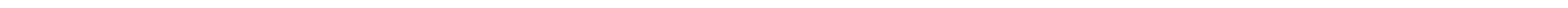# PyRated

a python package for rate distortion theory
Participants: Sarah Marzen, Simon DeDeo.

Rate-distortion theory is about lossy compression. It tells you how to discard information about the world in the presence of bandwidth constraints. It provides a framework for describing how optimal perception must degrade when resources are limited. For that reason, it can be used to think about basic problems in the biology and cognitive science of perception in new ways.

PyRated is a very simple Python package to do the most basic calculation in rate-distortion theory: the determination of the “codebook” and the transmission rate R, given a utility function (distortion matrix) and a Lagrange multiplier beta. It was written to do the calculations in our paper “The evolution of lossy compression”, where we introduce rate-distortion theory and also draw attention to a number of other recent papers that put this theorem from engineering into the biological and cognitive sciences. Those in psychology should take a look at a recent Cognition paper by Cris R. Sims at Drexel, “Rate–distortion theory and human perception”, which talks about rate-distortion theory in the context of bounded rationality, and provides an R package, RateDistortion.

If you use PyRated for anything cool, please let us know!

1. Introduction
2. Examples
3. BibTex for citation

In the simplest case, there are N possible states of the world, and an organism deterministically tracks all of them with N internal symbols; when the world is in state Xi, the organism is in state Yi. But what happens when the organism can’t gain enough information from the environment? Or, what happens when it can’t transmit the information from its sensory apparatus further back to higher-level processing because of capacity limits? In these situations, it’s natural to ask about rate-limited perception.

Rate-distortion theory allows you to talk about how an organism with bandwidth r can handle an environment with bandwidth r’ , where r’ > r. In particular, if you tell it how bad different types of confusions are, it will find the optimal transmission codebook that an organism can achieve, minimizing the costly errors without demanding too much transmission. When an organism’s bandwidth is too small, it can no longer map Xi to Yi exactly, but this codebook will do its best given the constraint on transmission rate.

More specifically, define a “distortion matrix” d, where d(i,j) is the cost of confusing environmental state i with environmental state j (e.g., 1 could be “there’s a snake”, 2 could be “there’s a garden hose”, and d(1,2) the cost of thinking something is a garden hose when it’s really a snake). Define also a distribution over environmental states, p, where p(i) is the probability of state i actually happening. Then the trick is to find an optimal encoding p(i|j)—the probability of sensing state i given that j is the true state—that gives the lowest distortion (cost), while not going larger than some rate r. The rate r, it turns out, is best encoded in terms of a conversion factor, beta, that tells you how costly mistakes are compared to bandwidth.

Then, the PyRated function getFeatures takes d(i,j) and p(i), and the conversion factor beta, and returns p(i|j) and bandwidth, r.

## Documentation

Here’s an example:

Python 2.7.13 (default, Dec 18 2016, 05:35:35)
[GCC 4.2.1 Compatible Apple LLVM 7.3.0 (clang-703.0.31)] on darwin
>>> from PyRated import *
>>> n=4
>>> d = makeDistortionMatrix(n,'exponential',1,1)

Make a distortion matrix for a world with four states, with an exponential distribution for the off-diagonals, offset by dmin=1

>>> d
array([[ 0. , 1.76873995, 1.75207931, 1.9652514 ],
[ 1.85139614, 0. , 1.22714042, 1.3826312 ],
[ 2.11482146, 1.00930419, 0. , 1.72105382],
[ 2.53243548, 1.40448744, 3.45731636, 0. ]])

Looking like a harsh world out there, with >1 penalties if you guess wrong.

>>> p = np.ones(n)/(n+0.0)
>>> pguess = np.ones(shape=d.shape)

Set up an environment with a uniform distribution over input states, and begin with the perfect codebook as an initial guess.

>>> pcode, R, D = getFeatures(p,d,0.75,pguess)

Run the codebook finder, with a beta value of 0.75.

>>> pcode
array([[ 0.59892252, 0.10564172, 0.13269154, 0.16987105],
[ 0.24790696, 0.66055256, 0.32642486, 0.43636244],
[ 0.13215798, 0.20126291, 0.53222621, 0.2198993 ],
[ 0.02101254, 0.03254281, 0.00865739, 0.1738672 ]])

Codebook looking a little sloppy, guys! State one, for example, has a 25% chance of being miscoded as state two. But it’s not crazy. Notice that our codebook is careful not to get that ~3.45 penalty for guessing state four if it’s actually state three. What’s our average penalty?

>>> D
0.80149609440157099

Non-zero, as expected. I hope we’re at least saving on transmission costs! There are two bits of information in the environment, but how many are we transmitting back?

>>> R
0.24311837254730873

Not bad. For perfect fidelity, we’d need R=2, but by accepting an average penalty of 0.8, we can transmit almost 90% less. Let’s try a really strict value of beta (equivalent to relaxing transmission penalties) of 100:

>>> pcode, R, D = getFeatures(p,d,100,pguess)
>>> D
3.7020138946730403e-45

Super hi-fi!

>>> R
2.0

But, yes, we have to transmit everything. Finally, let’s plot the R(D) curve itself (the basic task needed to reproduce the figures in the paper).

>>> betas, R, D = RD_curve(n,10,'exponential',1,1)
>>> pl.plot(D,R,'--.')
[matplotlib.lines.Line2D object at 0x116d59b10]
>>> pl.show()## BibTeX

@Article{marzen2017evolution,
title={The evolution of lossy compression},
author={Marzen, Sarah E and DeDeo, Simon},
journal={Journal of The Royal Society Interface},
volume={14},
number={130},
pages={20170166},
year={2017},
publisher={The Royal Society}
}